Linear Discriminant Analysis¶

Linear Discriminant Analysis are statistical analysis methods to find a linear combination of features for separating observations in two classes.

Note: Please refer to Multi-class Linear Discriminant Analysis for methods that can discriminate between multiple classes.

Overview of LDA¶

Suppose the samples in the positive and negative classes respectively with means: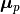and, and covariances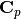and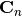. Then based on Fisher’s Linear Discriminant Criteria, the optimal projection direction can be expressed as: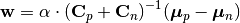Here α is an arbitrary non-negative coefficient.

Linear Discriminant¶

A linear discriminant functional can be written as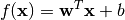Here, w is the coefficient vector, and b is the bias constant.

This package uses the LinearDiscriminant type, defined as below, to capture a linear discriminant functional:

immutable LinearDiscriminant <: Discriminant
w::Vector{Float64}
b::Float64
end

This type comes with several methods. Let f be an instance of LinearDiscriminant

length(f)

Get the length of the coefficient vector.

evaluate(f, x)

Evaluate the linear discriminant value, i.e w'x + b.

When x is a vector, it returns a real value; when x is a matrix with samples in columns, it returns a vector of length size(x, 2).

predict(f, x)

Make prediction. It returns true iff evaluate(f, x) is positive.

Data Analysis¶

The package provides several functions to perform Linear Discriminant Analysis.

ldacov(Cp, Cn, μp, μn)

Performs LDA given covariances and mean vectors.

Parameters: Cp – The covariance matrix of the positive class. Cn – The covariance matrix of the negative class. μp – The mean vector of the positive class. μn – The mean vector of the negative class. The resultant linear discriminant functional of type LinearDiscriminant.

Note: The coefficient vector is scaled such that w'μp + b = 1 and w'μn + b = -1.

ldacov(C, μp, μn)

Performs LDA given a covariance matrix and both mean vectors.

Parameters: C – The pooled covariane matrix (i.e (Cp + Cn)/2) μp – The mean vector of the positive class. μn – The mean vector of the negative class. The resultant linear discriminant functional of type LinearDiscriminant.

Note: The coefficient vector is scaled such that w'μp + b = 1 and w'μn + b = -1.

fit(LinearDiscriminant, Xp, Xn)

Performs LDA given both positive and negative samples.

Parameters: Xp – The sample matrix of the positive class. Xn – The sample matrix of the negative class. The resultant linear discriminant functional of type LinearDiscriminant.